# Gaussian process regression benchmarks¶

April 24, 2019 – hal14 (32 cores)

This page presents benchmarks in which we compare the performance of the Gaussian Process regression in Limbo against two other libraries: GPy (https://github.com/SheffieldML/GPy) and libGP (https://github.com/mblum/libgp).

The quality of the produced model is evaluated according to the Mean Squared Error (lower is better) with respect to the ground truth function. We also quantify the amount of time required by the different libraries to learn the model and to query it. In both cases, lower is better. The evaluations are replicated 30 times and for each replicate, all the variants (see below for the available variants) are using exactly the same data. The data are uniformly sampled and some noise is added (according to the variance of the data).

The comparison is done on 11 tasks to evaluate the performance of the libraries on functions of different complexity, and input/output spaces. The results show that the query time of Limbo’s Gaussian processes is several orders of magnitude better than the one of GPy and around twice better than libGP for a similar accuracy. The learning time of Limbo, which highly depends on the optimization algorithm chosen to optimize the hyper-parameters, is either equivalent or faster than the compared libraries.

It is important to note that the objective of the compared libraries are not necessarily the performance, but to provide baselines so that users know what to expect from Limbo and how it compares to other GP libraries. For instance, GPy is a python library with much more feature and designed to be easy to use. Moreover, GPy can achieve comparable performance with C++ libraries in the hyper-parameters optimization part because it utilizes numpy and scipy that is basically calling C code with MKL bindings (which is almost identical to what we are doing in Limbo).

## Variants¶

• GP-SE-Full-Rprop: Limbo with Squared Exponential kernel where the signal noise, signal variance and kernel lengthscales are optimized via Maximum Likelihood Estimation with the Rprop optimizer (default for limbo)
• GP-SE-Rprop: Limbo with Squared Exponential kernel where the signal variance and kernel lengthscales are optimized via Maximum Likelihood Estimation with the Rprop optimizer (default for limbo) and where the signal noise is not optimized but set to a default value: 0.01
• libGP-SE-Full: libGP with Squared Exponential kernel where the signal noise, signal variance and kernel lengthscales are optimized via Maximum Likelihood Estimation with the Rprop optimizer (the only one that libGP has)
• GPy: GPy with Squared Exponential kernel where the signal noise, signal variance and kernel lengthscales are optimized via Maximum Likelihood Estimation (with the L-BFGS-B optimizer — check scipy.optimize.fmin_l_bfgs_b=)

## Gramacy-Lee in 1D¶

Details about the function can be found here.

30 replicates## OTL Circuit in 6D¶

Details about the function can be found here.

30 replicates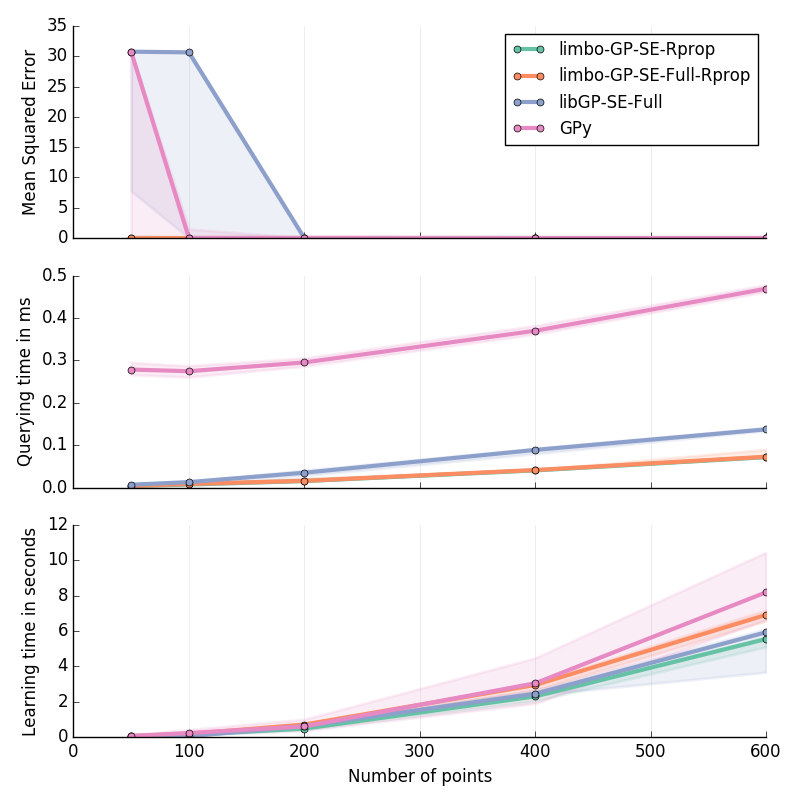## Piston Simulation in 7D¶

Details about the function can be found here.

30 replicates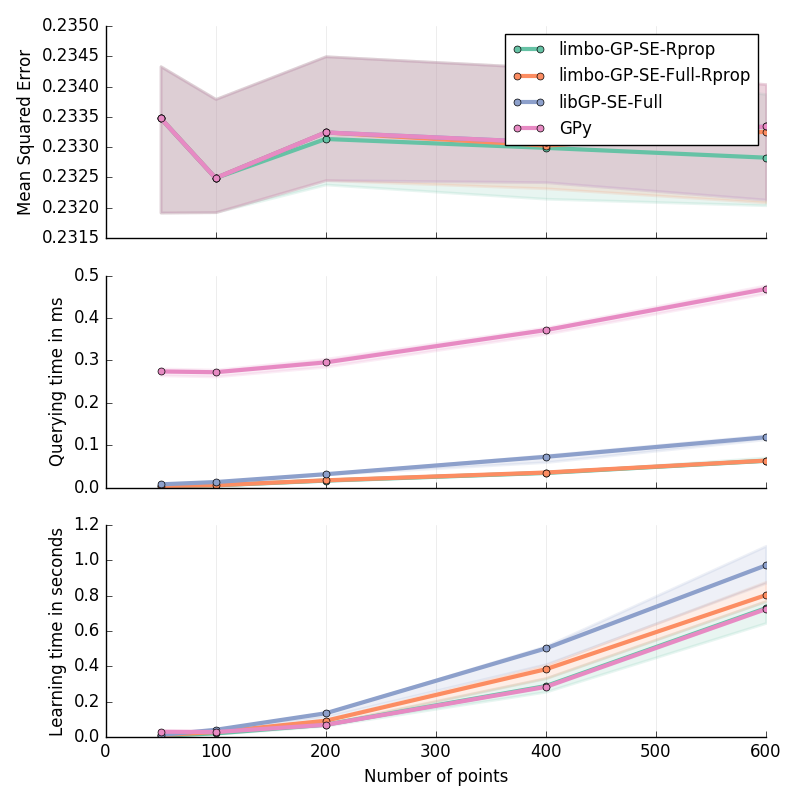## Planar Inverse Dynamics I in 6D¶

Approximation of the first motor’s torque in the inverse dynamics of a Planar 2D Arm. Details are given at the bottom of this page.

30 replicates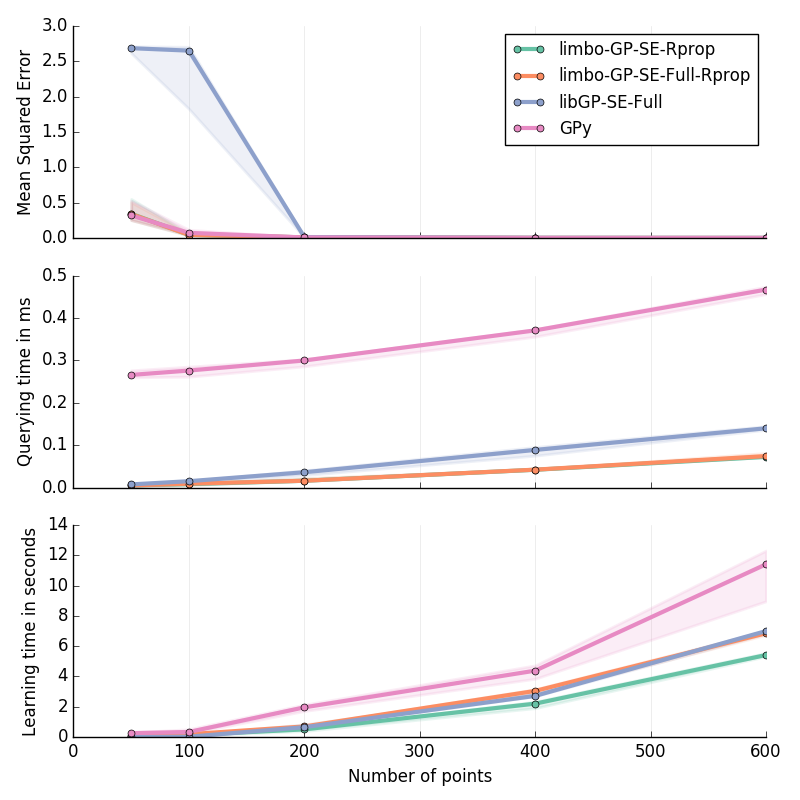## Planar Inverse Dynamics II in 6D¶

Approximation of the second motor’s torque in the inverse dynamics of a Planar 2D Arm. Details are given at the bottom of this page.

30 replicates## Rastrigin in 1D¶

Details about the function can be found here.

30 replicates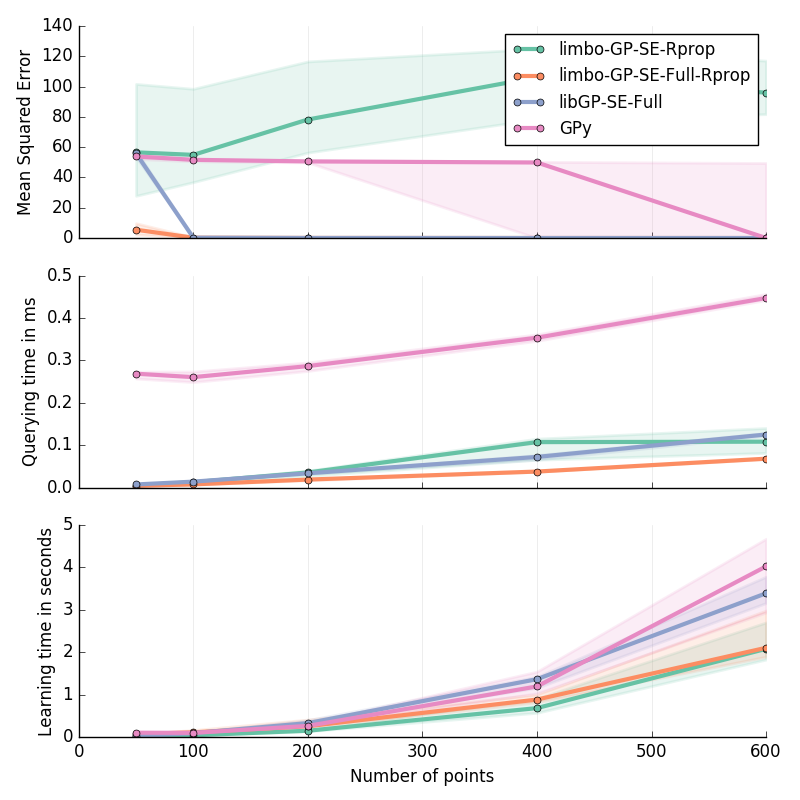## Rastrigin in 2D¶

Details about the function can be found here.

30 replicates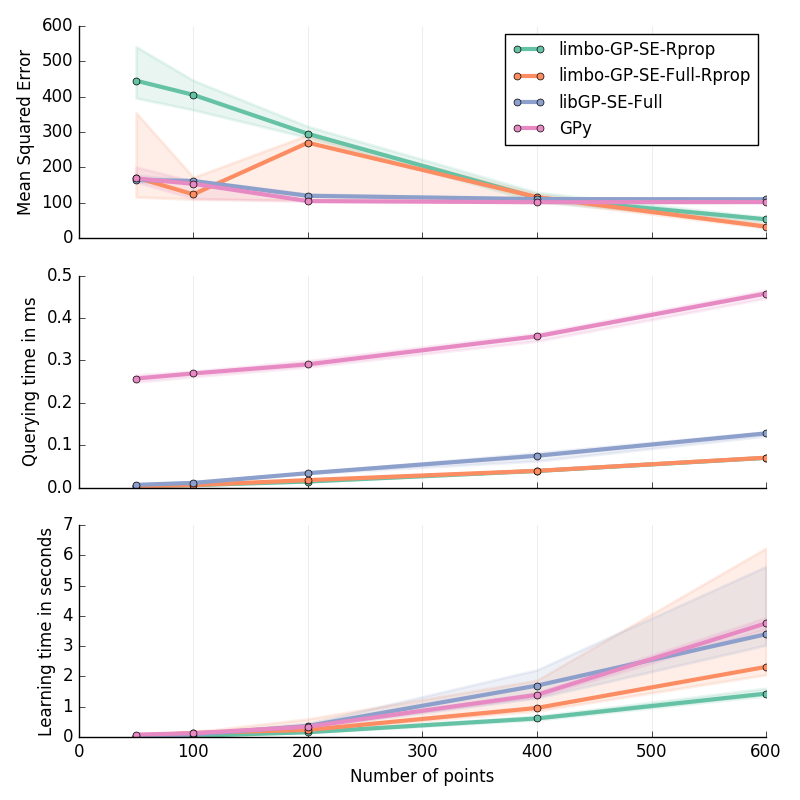## Rastrigin in 4D¶

Details about the function can be found here.

30 replicates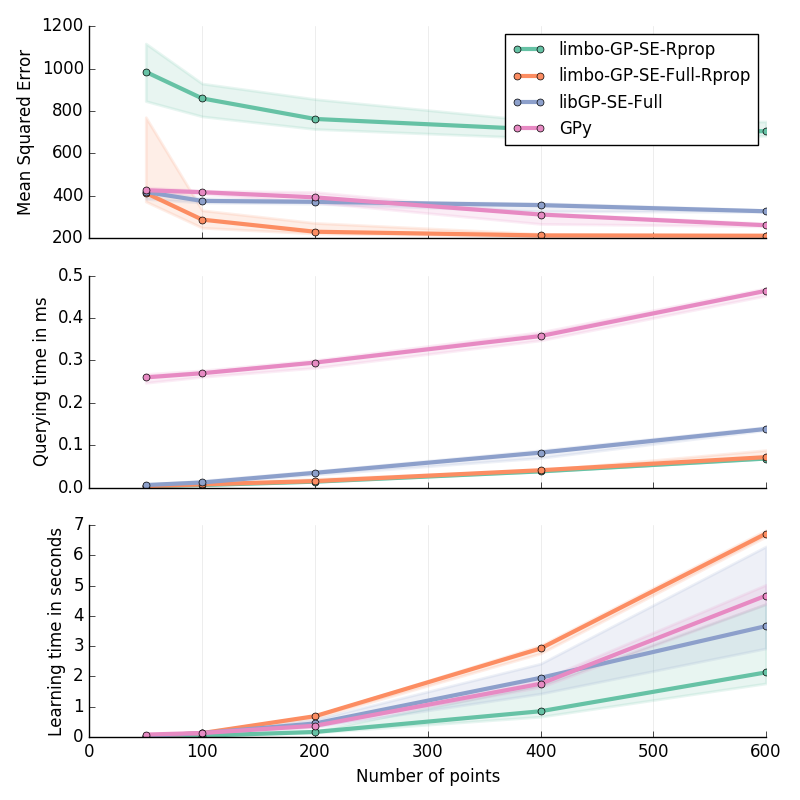## Rastrigin in 8D¶

Details about the function can be found here.

30 replicates## Robot Arm in 8D¶

Details about the function can be found here.

30 replicates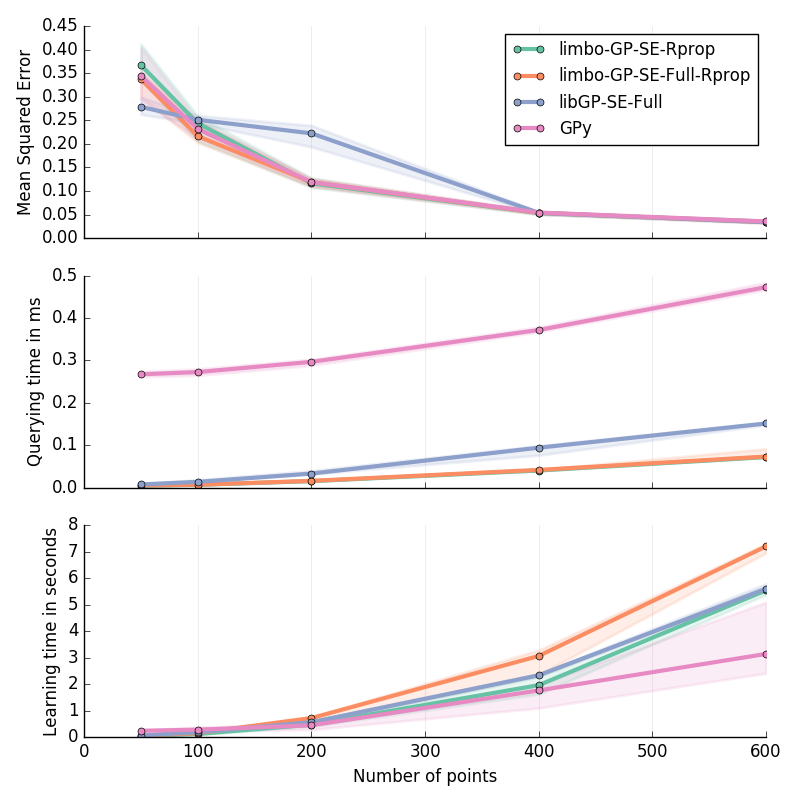## Step in 1D¶

Step function: for $$x\in[-2; 2]$$ :

$\begin{split}f(x) = \begin{cases} 0, &\mbox{if x<=0} \\ 1, &\mbox{otherwise} \end{cases}\end{split}$

30 replicates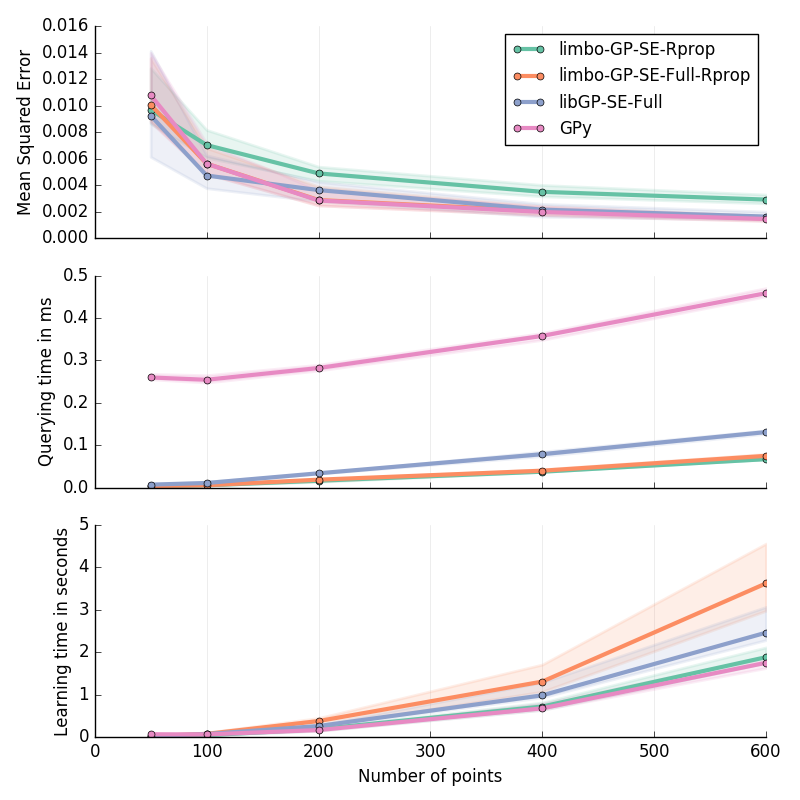Inverse Dynamics of a Planar 2D Arm (I & II): for $$\ddot{q}\in[-2\pi; 2\pi]^2$$; $$\dot{q}\in[-2\pi; 2\pi]^2$$; $$q\in[-pi; pi]^2$$

$\begin{split}\begin{gather} \tau (q,\dot{q},\ddot{q})=\textbf{M}(q)\ddot{q}+ \textbf{C}(q,\dot{q})\dot{q}\\ \textrm{where:}\\ \textbf{M}(q)=\begin{bmatrix} 0.2083 + 0.1250\cos(q_2)& 0.0417 + 0.0625\cos(q_2))\\ 0.0417 + 0.0625\cos(q_2)& 0.0417 \end{bmatrix} \\ \textbf{C}(q,\dot{q})=\begin{bmatrix} -0.0625 \sin(q_2) \dot{q}_2& -0.0625 \sin(q_2)(\dot{q}_1 + \dot{q}_2)\\ 0.0625 \sin(q_2)\dot{q}_1& 0 \end{bmatrix} \\ \end{gather}\end{split}$